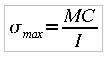# Moment of inertia and Stress

ricky_fusion
Hi..

Is there anyone can explain to me or give a link that explains about condition of using Mass Moment of inertia or Area moment of inertia to Maximum Stress??

http://en.wikipedia.org/wiki/Mass_moment_of_inertia" [Broken]

http://en.wikipedia.org/wiki/List_of_area_moments_of_inertia" [Broken]

As I knows, the stress formula is :As I knows, generally, I analysis a structure of Beam, etc, I used Area Moment of Inertia. But, I have a case which the knowing data is Mass moment of Inertia.

Thanks for your helps guys..Last edited by a moderator:

Homework Helper
Hi ricky_fusion!As I knows, generally, I analysis a structure of Beam, etc, I used Area Moment of Inertia. But, I have a case which the knowing data is Mass moment of Inertia.

moment of force = Stress times https://www.physicsforums.com/library.php?do=view_item&itemid=185" per displacement

moment of force = angular acceleration times mass moment

I don't understand how you can find the stress from the Mass moment of Inertia ("ordinary" https://www.physicsforums.com/library.php?do=view_item&itemid=31") …

can you show us this case in which the given data is mass moment?Last edited by a moderator:
ricky_fusion
Last edited by a moderator:
Homework Helper
I put the case here :

Hi Ricky!edit it to show what the question is!Last edited by a moderator:
ricky_fusion
Uupss...
Sorry I forgot to put put the question.The question is :
How much the maximum load should be put, so the beam is not broken?

Btw,
Let's continued discussed this thread in here :